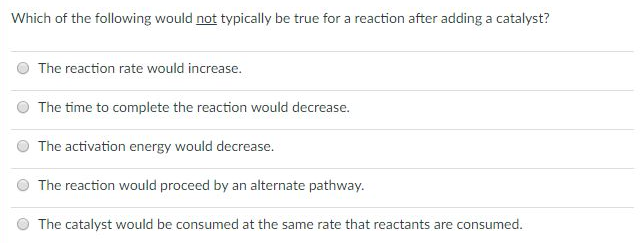# Problem: Which of the following would not typically be true for a reaction after adding a catalyst? a. The reaction rate would increase. b. The time to complete the reaction would decrease.c. The activation energy would decrease.d. The reaction would proceed by an alternate pathway.e. The catalyst would be consumed at the same rate that reactants are consumed.

###### FREE Expert Solution
80% (221 ratings)###### Problem Details

Which of the following would not typically be true for a reaction after adding a catalyst?

a. The reaction rate would increase.

b. The time to complete the reaction would decrease.

c. The activation energy would decrease.

d. The reaction would proceed by an alternate pathway.

e. The catalyst would be consumed at the same rate that reactants are consumed.